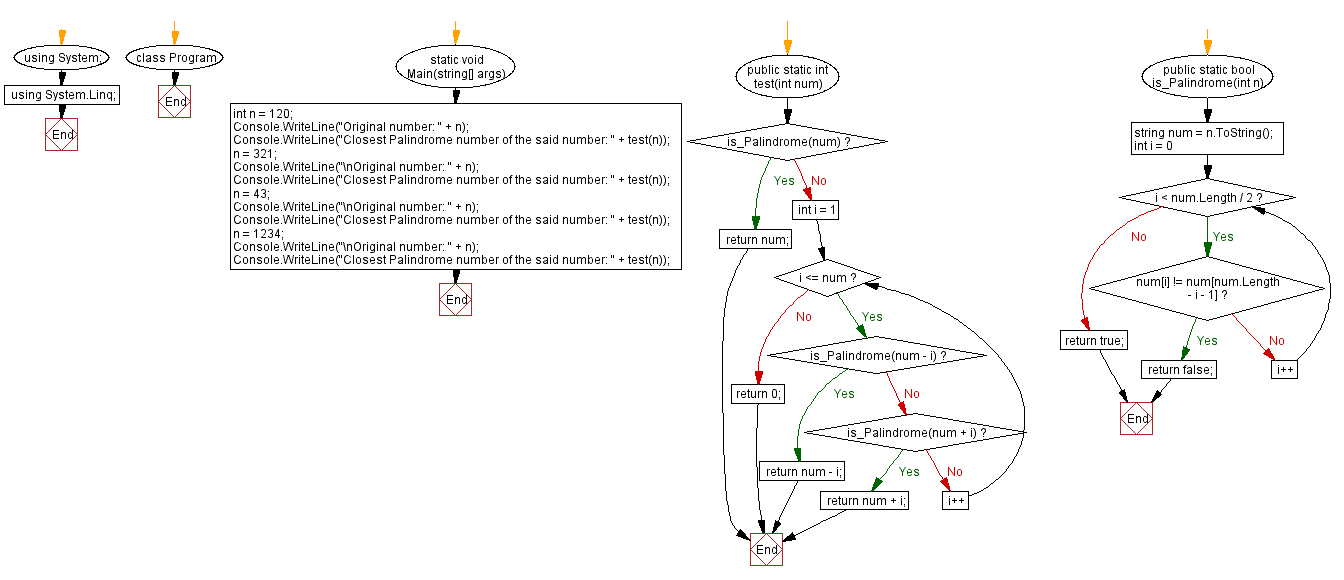﻿ C# - Closest Palindrome Number# C# Sharp Exercises: Closest Palindrome Number

## C# Sharp Math: Exercise-25 with Solution

Write a C# Sharp program to find the closest palindrome number of a given integer. If there are two palindrome numbers in absolute distance return the smaller number.

Sample Solution:

C# Sharp Code:

``````using System;
using System.Linq;
namespace exercises
{
class Program
{
static void Main(string[] args)
{
int n = 120;
Console.WriteLine("Original number: " + n);
Console.WriteLine("Closest Palindrome number of the said number: " + test(n));
n = 321;
Console.WriteLine("\nOriginal number: " + n);
Console.WriteLine("Closest Palindrome number of the said number: " + test(n));
n = 43;
Console.WriteLine("\nOriginal number: " + n);
Console.WriteLine("Closest Palindrome number of the said number: " + test(n));
n = 1234;
Console.WriteLine("\nOriginal number: " + n);
Console.WriteLine("Closest Palindrome number of the said number: " + test(n));
}
public static int test(int num)
{
if (is_Palindrome(num)) return num;
for (int i = 1; i <= num; i++)
{
if (is_Palindrome(num - i)) return num - i;
if (is_Palindrome(num + i)) return num + i;
}
return 0;
}

public static bool is_Palindrome(int n)
{
string num = n.ToString();

for (int i = 0; i < num.Length / 2; i++)
{
if (num[i] != num[num.Length - i - 1])
return false;
}
return true;
}
}
}
```
```

Sample Output:

```Original number: 120
Closest Palindrome number of the said number: 121

Original number: 321
Closest Palindrome number of the said number: 323

Original number: 43
Closest Palindrome number of the said number: 44

Original number: 1234
Closest Palindrome number of the said number: 1221

```

Flowchart:C# Sharp Code Editor:

Improve this sample solution and post your code through Disqus

Next: Check a number is uban number or not.

What is the difficulty level of this exercise?

Test your Programming skills with w3resource's quiz.

﻿